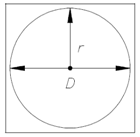## Circles Assignment Help

Circles:

A circle is a plane curve that is equidistant from the center, as shown in Figure. The length of the perimeter of a circle is known as the circumference. A radius (r) of a circle is a line segment which joins the center of a circle with any point on its circumference. The diameter (D) of a circle is a line segment connecting two points of the circle through the center.  The area of a circle is computed using the following formula:A =      πr2

The circumference which is given above of a circle is computed using the subsequent formula:

C = 2πr

or

C =      D

Pi (π) is a theoretical number, approximately 22/7/3.141592654, representing the ratio of the circumference to the diameter of a circle. The scientific calculator makes this simple by designating a key for determining.

An area of a circle is always expressed in square units, and the perimeter of a circle is always expressed in the original units.